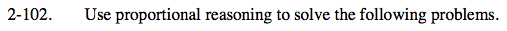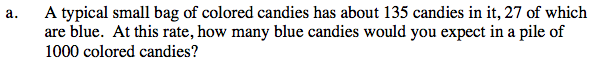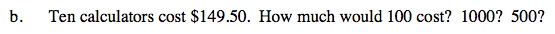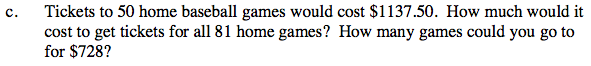### Home > CAAC > Chapter 2 > Lesson 2.2.2 > Problem2-102

2-102.Use proportions to set up the problem.

$\frac{27\text{ blue}}{135\text{total}} = \frac{n}{1000\text{ total}}$

$27\cdot 1000=135 \cdot n$

$\frac{27000}{135}=\frac{135n}{135}$

n = 200Use proportions to set up the problem.

$\frac{10}{149.50}=\frac{100}{n}$

Multiply by a fraction equal to 1 in order to make the equivalent proportions have exactly similar numbers.

$\frac{10}{149.50}\cdot \frac{10}{10}=\frac{100}{n}$

Solve for n by multiplying 149.50 by 10.

n = \$1495Use the strategy seen in part (a).